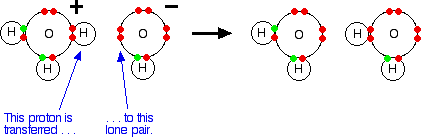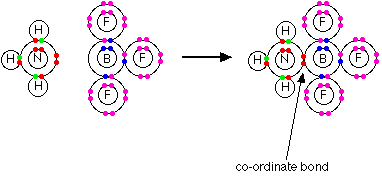# 1. Theories of Acids and Bases

$$\newcommand{\vecs}{\overset { \rightharpoonup} {\mathbf{#1}} }$$ $$\newcommand{\vecd}{\overset{-\!-\!\rightharpoonup}{\vphantom{a}\smash {#1}}}$$$$\newcommand{\id}{\mathrm{id}}$$ $$\newcommand{\Span}{\mathrm{span}}$$ $$\newcommand{\kernel}{\mathrm{null}\,}$$ $$\newcommand{\range}{\mathrm{range}\,}$$ $$\newcommand{\RealPart}{\mathrm{Re}}$$ $$\newcommand{\ImaginaryPart}{\mathrm{Im}}$$ $$\newcommand{\Argument}{\mathrm{Arg}}$$ $$\newcommand{\norm}{\| #1 \|}$$ $$\newcommand{\inner}{\langle #1, #2 \rangle}$$ $$\newcommand{\Span}{\mathrm{span}}$$ $$\newcommand{\id}{\mathrm{id}}$$ $$\newcommand{\Span}{\mathrm{span}}$$ $$\newcommand{\kernel}{\mathrm{null}\,}$$ $$\newcommand{\range}{\mathrm{range}\,}$$ $$\newcommand{\RealPart}{\mathrm{Re}}$$ $$\newcommand{\ImaginaryPart}{\mathrm{Im}}$$ $$\newcommand{\Argument}{\mathrm{Arg}}$$ $$\newcommand{\norm}{\| #1 \|}$$ $$\newcommand{\inner}{\langle #1, #2 \rangle}$$ $$\newcommand{\Span}{\mathrm{span}}$$$$\newcommand{\AA}{\unicode[.8,0]{x212B}}$$

This Module describes the Arrhenius, Brønsted-Lowry, and Lewis theories of acids and bases, and explains the relationships between them. It also explains the concept of a conjugate pair - an acid and its conjugate base, or a base and its conjugate acid.

## The Arrhenius Theory of acids and bases

• Acids are substances that produce hydrogen ions in solution.
• Bases are substances that produce hydroxide ions in solution.

Neutralization happens because hydrogen ions and hydroxide ions react to produce water.

$H^+_{(aq)} + OH^-{(aq)} \rightarrow H_2O_{(l)}$

Hydrochloric acid is neutralized by both sodium hydroxide solution and ammonia solution. In both cases, you get a colorless solution which you can crystallize to get a white salt - either sodium chloride or ammonium chloride. These are clearly very similar reactions. The full equations are:

$NaOH_{(aq)} + HCl_{(aq)} \rightarrow NaCl_{(aq)} + H_2O_{(l)}$

$NH_{3(aq)} + HCl_{(aq)} \rightarrow NH_4Cl_{(aq)}$

In the sodium hydroxide case, hydrogen ions from the acid are reacting with hydroxide ions from the sodium hydroxide - in line with the Arrhenius theory. However, in the ammonia case, there do not appear to be any hydroxide ions! You can get around this by saying that the ammonia reacts with the water it is dissolved in to produce ammonium ions and hydroxide ions:

$NH_{3(aq)} + H_2O_{(l)} \rightleftharpoons NH^+_{4(aq)} + OH_{(aq)}^-$

This is a reversible reaction, and in a typical dilute ammonia solution, about 99% of the ammonia remains as ammonia molecules. Nevertheless, there are hydroxide ions there, and we can squeeze this into the Arrhenius theory. However, this same reaction also happens between ammonia gas and hydrogen chloride gas.

$NH_{3(g)} + HCl_{(g)} \rightarrow NH_4Cl_{(s)}$

In this case, there are no hydrogen ions or hydroxide ions in solution - because there is not any solution. The Arrhenius theory would not count this as an acid-base reaction, despite the fact that it is producing the same product as when the two substances were in solution.

## The Brønsted-Lowry Theory of acids and bases

• An acid is a proton (hydrogen ion) donor.
• A base is a proton (hydrogen ion) acceptor.

The Brønsted-Lowry theory does not go against the Arrhenius theory in any way - it just adds to it. Hydroxide ions are still bases because they accept hydrogen ions from acids and form water. An acid produces hydrogen ions in solution because it reacts with the water molecules by giving a proton to them.

When hydrogen chloride gas dissolves in water to produce hydrochloric acid, the hydrogen chloride molecule gives a proton (a hydrogen ion) to a water molecule. A coordinate (dative covalent) bond is formed between one of the lone pairs on the oxygen and the hydrogen from the $$HCl$$. Hydronium ions, $$H_3O^+_{(aq)}$$, are pr oduced.$H_2O + HCl \rightarrow H_3O^+ +Cl^-$

When an acid in solution reacts with a base, what is actually functioning as the acid is the hydronium ion. For example, a proton is transferred from a hydronium ion to a hydroxide ion to make water.

$H_3O^+_{(aq)} + OH^-_{(aq)} \rightarrow 2H_2O_{(l)}$

Showing the electrons, but leaving out the inner ones:It is important to realize that whenever you talk about hydrogen ions in solution, $$H^+_{(aq)}$$, wha t you are actually talking about are hydronium ions.

### The hydrogen chloride / ammonia problem

This is no longer a problem using the Brønsted-Lowry theory. Whether you are talking about the reaction in solution or in the gas state, ammonia is a base because it accepts a proton (a hydrogen ion). The hydrogen becomes attached to the lone pair on the nitrogen of the ammonia via a co-ordinate bond.If it is in solution, the ammonia accepts a proton from a hydronium ion:

$NH_{3 (aq)} + H_3O_{(aq)}^+ \rightarrow NH^+_{4(aq)} + H_2O_{(l)}$

If the reaction occurs in the gas state, the ammonia accepts a proton directly from the hydrogen chloride:

$NH_{3 (g)} + HCl_{(g)} \rightarrow NH_4Cl_{(s)}$

Either way, the ammonia acts as a base by accepting a hydrogen ion from an acid.

## Conjugate pairs

When hydrogen chloride dissolves in water, almost 100% of it reacts with the water to produce hydronium ions and chloride ions. Hydrogen chloride is a strong acid, and we tend to write this as a one-way reaction:

$H_2O + HCl \rightarrow H_3O^+ + Cl^-$

In fact, the reaction between HCl and water is reversible, but only to a very minor extent. To generalize, consider an acid $$HA$$, and think of the reaction as being reversible.

$HA + H_2O \rightleftharpoons H_3O^+ + A^-$

• The $$HA$$ is an acid because it is donating a proton (hydrogen ion) to the water.
• The water is a base because it is accepting a proton from the $$HA$$.

However, there is also a back reaction between the hydronium ion and the $$A^-$$ i on:

• The $$H_3O^+$$ is an acid because it is donating a proton (hydrogen ion) to the $$A^-$$ ion.
• The $$A^-$$ ion is a base because it is accepting a proton from the $$H_3O^+$$.

The reversible reaction contains two acids and two bases. We think of them in pairs, called conjugate pairs.When the acid, $$HA$$, loses a proton it forms a base, $$A^-$$, which can a ccept a proton back again to refom the acid, $$HA$$. These two are a conjugate pair. Members of a conjugate pair differ from each other by the presence or absence of the transferable hydrogen ion.

• If you are thinking about $$HA$$ as the acid, then $$A^-$$ is its c onjugate base.
• If you are thinking about $$A^-$$ as the base, then $$HA$$ is its con jugate acid.

The water and the hydronium ion are also a conjugate pair. Thinking of the water as a base, the hydronium ion is its conjugate acid because it has the extra hydrogen ion which it can give away again. Thinking about the hydronium ion as an acid, then water is its conjugate base. The water can accept a hydrogen ion back again to reform the hydronium ion.

## A second example of conjugate pairs

This is the reaction between ammonia and water that we looked at earlier:Think first about the forward reaction. Ammonia is a base because it is accepting hydrogen ions from the water. The ammonium ion is its conjugate acid - it can release that hydrogen ion again to reform the ammonia.

The water is acting as an acid, and its conjugate base is the hydroxide ion. The hydroxide ion can accept a hydrogen ion to reform the water. Looking at it from the other side, the ammonium ion is an acid, and ammonia is its conjugate base. The hydroxide ion is a base and water is its conjugate acid.

## Amphoteric substances

You may possibly have noticed (although probably not!) that in one of the last two examples, water was acting as a base, whereas in the other one it was acting as an acid. A substance which can act as either an acid or a base is described as being amphoteric.## The Lewis Theory of acids and bases

This theory extends well beyond the things you normally think of as acids and bases.

• An acid is an electron pair acceptor.
• A base is an electron pair donor.

### Lewis bases

It is easiest to see the relationship by looking at exactly what Brønsted-Lowry bases do when they accept hydrogen ions. Three Brønsted-Lowry bases we've looked at are hydroxide ions, ammonia and water, and they are typical of all the rest.The Brønsted-Lowry theory says that they are acting as bases because they are combining with hydrogen ions. The reason they are combining with hydrogen ions is that they have lone pairs of electrons - which is what the Lewis theory says. The two are entirely consistent. So how does this extend the concept of a base? At the moment it doesn't - it just looks at it from a different angle.

But what about other similar reactions of ammonia or water, for example? On the Lewis theory, any reaction in which the ammonia or water used their lone pairs of electrons to form a co-ordinate bond would be counted as them acting as a base. Here is a reaction which you will find talked about on the page dealing with co-ordinate bonding. Ammonia reacts with BF3 by using its lone pair to form a co-ordinate bond with the empty orbital on the boron.As far as the ammonia is concerned, it is behaving exactly the same as when it reacts with a hydrogen ion - it is using its lone pair to form a co-ordinate bond. If you are going to describe it as a base in one case, it makes sense to describe it as one in the other case as well.

### Lewis acids

Lewis acids are electron pair acceptors. In the above example, the BF3 is acting as the Lewis acid by accepting the nitrogen's lone pair. On the Brønsted-Lowry theory, the BF3 has nothing remotely acidic about it. This is an extension of the term acid well beyond any common use.

What about more obviously acid-base reactions - like, for example, the reaction between ammonia and hydrogen chloride gas?

$NH_{3(g)} + HCl_{g)} \rightarrow NH^+_{4(s)} + Cl^-_{(s)}$

What exactly is accepting the lone pair of electrons on the nitrogen. Textbooks often write this as if the ammonia is donating its lone pair to a hydrogen ion - a simple proton with no electrons around it. That is misleading! You don't usually get free hydrogen ions in chemical systems. They are so reactive that they are always attached to something else. There aren't any uncombined hydrogen ions in HCl.

There isn't an empty orbital anywhere on the HCl which can accept a pair of electrons. Why, then, is the HCl a Lewis acid? Chlorine is more electronegative than hydrogen, and that means that the hydrogen chloride will be a polar molecule. The electrons in the hydrogen-chlorine bond will be attracted towards the chlorine end, leaving the hydrogen slightly positive and the chlorine slightly negative.The lone pair on the nitrogen of an ammonia molecule is attracted to the slightly positive hydrogen atom in the HCl. As it approaches it, the electrons in the hydrogen-chlorine bond are repelled still further towards the chlorine. Eventually, a coordinate bond is formed between the nitrogen and the hydrogen, and the chlorine breaks away as a chloride ion.

This is best shown using the "curly arrow" notation commonly used in organic reaction mechanisms.The whole HCl molecule is acting as a Lewis acid. It is accepting a pair of electrons from the ammonia, and in the process it breaks up. Lewis acids don't necessarily have to have an existing empty orbital.

##### Note

All you need to remember for Lewis acids and bases is:

• A Lewis acid is an electron pair acceptor.
• A Lewis base is an electron pair donor.

For all general purposes, stick with the Brønsted-Lowry theory.

This page titled 1. Theories of Acids and Bases is shared under a CC BY-NC 4.0 license and was authored, remixed, and/or curated by Jim Clark.